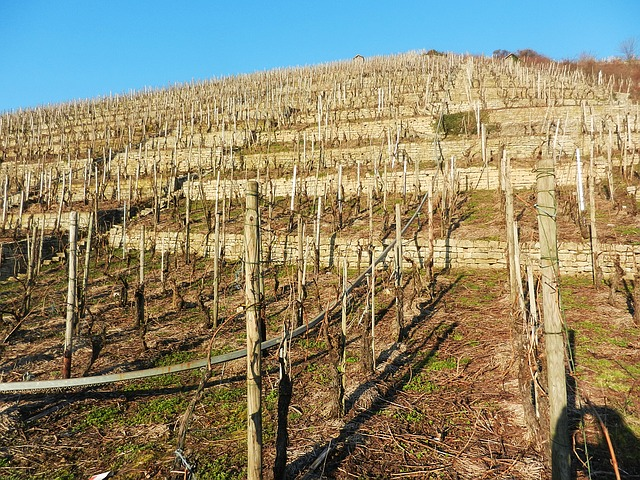Feb 26, 2016

Feb 26, 2016

Feb 26, 2016

# General Considerations and Assumptions in the Soil Stability AnalysisThere are three distinct parts to an analysis of the stability of a slope. They are:

### 1. Testing of samples to determine the cohesion and angle of internal friction

If the analysis is for a natural slope, it is essential that the sample be undisturbed. In such important respects as rate of shear application and state of initial consolidation, the condition of testing must represent as closely as possible the most unfavorable conditions ever likely to occur in the actual slope.

### 2. The study of items which are known to enter but which cannot be accounted for in the computations

The most important of such items is progressive cracking which will start at the top of the slope where the soil is in tension, and aided by water pressure, may progress to considerable depth. In addition, there are the effects of the non‐homogeneous nature of the typical soil and other variations from the ideal conditions which must be assumed.

### 3. Computation

If a slope is to fail along a surface, all the shearing strength must be overcome along that surface which then becomes a surface of rupture. Any one such as ABC in Fig. 2 (b) represents one of an infinite number of possible traces on which failure might occur.

Also See: GeoTechnical Engineering

It is assumed that the problem is two dimensional, which theoretically requires a long length of slope normal to the section.
The shear strength of soil is assumed to follow Mohr Coulomb's law Τ or s = c̕+ σ' tan φ̕

Where, c' ‐ effective unit cohesion

σ'= effective normal stress on the surface of rupture = (σ ‐ u)

σ ‐ total normal stress on the surface of rupture

u ‐ pore water pressure on the surface of rupture

φ̕= effective angle of internal friction.

The item of great importance is the loss of shearing strength which many clays show when subjected to a large shearing strain. The stress‐strain curves for such clay show the stress rising with increasing strain to a maximum value, after which it decreases and approaches an ultimate  value which may be much less than the maximum. Since a rupture surface tends to develop progressively rather than with all the points at the same state of strain, it is generally the ultimate value that should be used for the shearing strength rather than the maximum value.